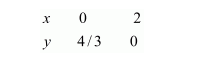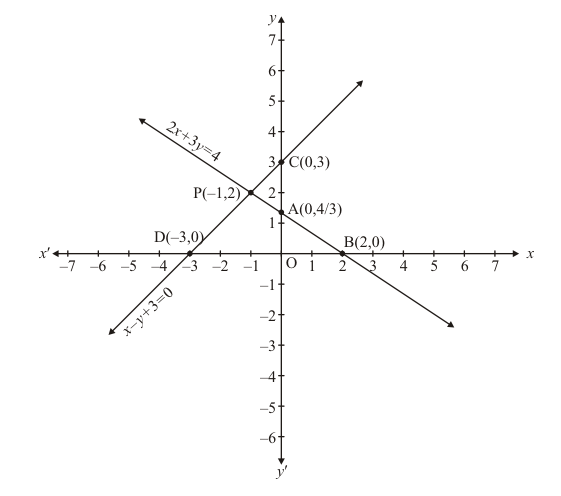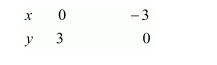# Solve the following systems of equations graphically:`
Question:

Solve the following systems of equations graphically:

$2 x+3 y=4$

$x-y+3=0$

Solution:

The given equations are:

$2 x+3 y=4$ $.(i)$

$x-y+3=0$ ..(ii)

Putting $x=0$ in equation $(i)$, we get:

$\Rightarrow 2 \times 0+3 y=4$

$\Rightarrow y=4 / 3$

$x=0, \quad y=4 / 3$

Putting $y=0$ in equation $(i,$, we get:

$\Rightarrow 2 x+3 \times 0=4$

$\Rightarrow x=2$

$x=2, \quad y=0$

Use the following table to draw the graph.Draw the graph by plotting the two points $A(0,4 / 3)$ and $B(2,0)$ from tableGraph of the equation....(ii):

$x-y=-3$ .(ii)

Putting $x=0$ in equation (ii) we get:

$\Rightarrow 0-y=-3$

$\Rightarrow y=3$

$x=0, \quad y=3$

Putting $y=0$ in equation $(i i)$, we get:

$\Rightarrow x-0=-3$

$\Rightarrow x=-3$

$x=-3, \quad y=0$

Use the following table to draw the graph.Draw the graph by plotting the two points $C(0,3)$ and $D(-3,0)$ from table

The two lines intersect at points $P(-1,2)$.

Hence, $x=-1$ and $y=2$ is the solution.